## Constructing a Yield Curve in SQL Server

Sep 26

Written by: Charles Flock
9/26/2016 1:48 PMThe XLeratorDB table-valued function SWAPCURVE takes cash, futures, and swaps rates as input and returns discount factors and continuously compounded zero coupon rates which can then be used in a variety of sophisticated financial calculations. In this article we take an in-depth look at the mechanics of SWAPCURVE calculation using SQL and other XLeratorDB functions to explain where the numbers come from. These alternative calculations are designed to be explanatory and not as a substitute for the SWAPCURVE function which will be, by far, the fastest and easiest way to generate the curve values.
Here are some rates as at 09-Sep-2016.
Cash Rates

 O/N 0.42211% 1W 0.44522% 1M 0.51822% 2M 0.66467% 3M 0.84544% 6M 1.54033%

Futures Prices

 16-Dec 99.045 17-Mar 99.01 17-Jun 98.965 17-Sep 98.93 17-Dec 98.89

Swap Rates

 1Y 0.9200% 2Y 1.0100% 3Y 1.0900% 4Y 1.0800% 5Y 1.2200% 7Y 1.3500% 10Y 1.5000% 15Y 1.4700% 30Y 1.8200%

### Picking a starting point

At its center, building a yield a curve is a time-value-of-money (TVM) exercise. TVM calculations either explicitly or implicitly require the calculation of discount factors. The basic equation for the calculation of the discount factor is: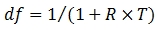Where
df = discount factor
R = interest rate
T = time (in year)
There are two inputs into the equation: a rate and the time associated with that rate. In order to calculate the discount factor we need to establish the origination point in time for all the calculations, which in this case is 2016-09-09.
DECLARE @TODAY as date = CAST('2016-09-09' as datetime)

### Calculating the spot date

In constructing the swap curve, we have to account for the market conventions regarding rate quotes. Generally speaking, maturity dates are quoted as a number of months (cash markets) or years (swaps markets) from the spot date. The spot date is generally 2 business days from the current date. Thus the spot date for our example would be 2 business days after 2016-09-09; 2016-09-13. The BUSINESSDATE function will automatically calculate this.
DECLARE @SPOT as date = wct.BUSINESSDATE(@TODAY,'D',2,'M','')

### Calculating the maturity dates

Maturity dates are calculated with respect to the spot date. Thus the cash rate for 1 month maturity is 1 month from the spot date. The 2-year swap rate is 2 years from the spot date. If the maturity date falls on a non-business date, then the date is advanced to the next business day unless that next business day is in the subsequent month in which case the date retreats to the previous business date. The TENOR2DATE function automatically calculates these dates for us.
The overnight rate is the rate from the processing date to the next business date. The tom-next rate is the rate from the next business date to the spot date. The spot-next rate is the 1-day rate from the spot date.

### Calculating the futures delivery dates

For futures, the date that is quoted is the delivery date of the contract, not the maturity date. The ED_FUT2DATE automatically converts the conventional Euro futures delivery code into delivery dates. The futures delivery dates are calculated with respect to the processing date rather than spot date.

### Put the data into a table

Here's some simple SQL that takes the market data and populates a table called #curves which will become the input for constructing the yield curve. Note that the futures prices are converted interest rates using the formula (100 – price) / 100.
SELECT
CASE iType
WHEN 'F' THEN wct.ED_FUT2DATE(mDate, @TODAY)
ELSE wct.TENOR2DATE(mDate,@TODAY,@SPOT,'')
END as mDate,
CASE iType
WHEN 'F' THEN (100 - cRate) / 100
ELSE cRate
END as cRate,
iType
INTO
#curves
FROM (VALUES
('ON', 0.0042211, 'C'),
('1W', 0.0044522, 'C'),
('1M', 0.0051822, 'C'),
('2M', 0.0066467, 'C'),
('3M', 0.0084544, 'C'),
('6M', 0.0154033, 'C'),
('1Y', 0.0092, 'S'),
('2Y', 0.0101, 'S'),
('3Y', 0.0109, 'S'),
('4Y', 0.0108, 'S'),
('5Y', 0.0122, 'S'),
('7Y', 0.0135, 'S'),
('10Y', 0.0150, 'S'),
('15Y', 0.0147, 'S'),
('30Y', 0.0182, 'S'),
('Z6', 99.0450, 'F'),
('H7', 99.0100, 'F'),
('M7', 98.9650, 'F'),
('U7', 98.9300, 'F'),
('Z7', 98.8900, 'F')
)n(mDate, cRate, iType)
This is what the #curves table should look like.
Remember, the easiest way to create the curve is to use the SWAPCURVE table-valued function!
SELECT
*
FROM
wct.SWAPCURVE(
'SELECT * FROM #curves'    --@InputData_RangeQuery
,@TODAY                     --@StartDate
,2                          --@Frequency
,@SPOT                      --@SpotDate
,2                          --@CashBasis
,2                          --@FuturesBasis
,2                          --@SwapsBasis
,'L'                        --@InterpMethod
,'M'                        --@DateRoll
,NULL                       --@Holidays
)
This produces the following result.

### Building the cash part of the curve

In this example, calculating the cash part of the curve (the part of the curve generated by the cash rates) is basically a 2-step process. First, calculate the discount factor from the processing date to the spot date. Then calculate the discount factor from the spot date to the individual maturity dates and then discount them from the spot date back to the processing date.

### Converting the overnight rate to a discount factor

We are going to create a table variable (@cash) for storing the cash rates and then put the overnight rate (which is the first cash rate that we have a quote for) into the table variable. We will use the XLeratorDB scalar function YEARFRAC to calculate the time (T) in years.
--Table variable for storing the result
DECLARE @cash as TABLE (
mDate datetime,
iType char(1),
df     float
)
--Put the overnight rate into the table
INSERT INTO
@cash
SELECT TOP 1
mdate,
iType,
1 /(1 + cRate * wct.YEARFRAC(@today,mdate,2))
FROM
#curves
WHERE
iType = 'C'

### Getting a rate for the spot date and converting it to a discount factor

The maturity dates for the cash instruments are quoted with respect to the spot date. In terms of the discount formula this means that the time value (T) associated with the rate (R) is the time from the spot date to the maturity date. Since we are interested in calculating the discount factor as of today rather than as of 2 business days in the future we need to have a discount factor for the spot date so that we can discount the cash discount factors from the spot date to the start date. In our example, there is no rate for the spot date, so we need infer one.
--Since there is no rate for the spot date we have to assume one
INSERT INTO
@cash
SELECT
@SPOT,
'I',
cRate,
1 /(1 + cRate * wct.YEARFRAC(@on,@spot,2)) * df
FROM
@cash
--Store the spot rate
DECLARE @df_spot as float =(SELECT df FROM @cash WHERE mdate = @SPOT)

### Converting all the other cash rates to discount factors

We have already calculated the discount factor as of the spot date. Building out the rest of the cash part of the curve requires calculating the discount factor for all the remaining cash instruments in the #curves table using the discount formula. Again, we use the YEARFRAC function to calculate the time (T) values.
Since the T values for these instruments are calculated from the spot date to the maturity date, the calculated discount factors need to be adjusted to account for the time from the start date to the spot date. Multiplying by the discount factor for the spot date gives us the discount factor from the start date to the maturity date of the cash instrument(s).
--Calculate the discount factors for the cash rates and put then into the table
INSERT INTO
@cash
SELECT
mDate
,'C' iType
,(1/(1+CRate*wct.YEARFRAC(@SPOT,mdate,2)))*@df_spot as df
FROM
#curves
WHERE
iType = 'C'
AND mDate > @SPOT
This is what the @cash table should look like.

### Calculating the discount factors for the futures strip

Eurodollar futures contracts (in this example) are basically cash rates with a future start date (the delivery date of the contract). The rate on the futures is a forward / forward rate with the value date equal to the delivery date and the maturity date 91 days thereafter. Even though each contract has a term of 91 days, for purposes of construction the curve we assume the term of contract runs from one delivery to the next so that there is no gap in the curve.
The calculation of the discount factor for each forward contract is essentially the same calculation as the discount factor for the cash instruments, 1 / (1+R*T) where R is the interest rate for the futures (100-price)/100 and T is the time in years from the delivery date to the next delivery date using the actual / 360 day-count convention. For use in the construction of the curve, however, these forward discount factors need to be adjusted back to the processing date.

### Getting the discount factor for the start of the futures strip

Mechanically this adjustment is actually quite simple. Calculate the interpolated discount factor as at the first delivery date and then calculate the cumulative product of the forward discount factors associated with the futures strip. In our implementation we use the DFINTERP function which performs discount factor interpolation.
--Table variable for storing futures strip result
DECLARE @futures as TABLE (
mDate datetime,
iType char(1),
df     float
)
--Determine when the futures strip commences
DECLARE @date_start_strip as datetime =(
SELECT TOP 1
mDate
FROM
#curves
WHERE
iType = 'F'
ORDER BY
mDate ASC
)
--Capture the interpolated rate for the start of the futures strip
DECLARE @df_start_strip as float =(
SELECT
wct.DFINTERP(c.mDate,c.df,@date_start_strip,@TODAY,'DF')
FROM
@cash c
)

### Calculating the discount factors after the first delivery date

Using some built-in SQL Server windowing functions and the XLeratorDB windowing function RunningPRODUCT, we can calculate the discount factors for the futures strip with a few lines of SQL.
--Put the discount factors for the futures strip into the table
INSERT INTO
@futures
SELECT
mDate,
CASE
WHEN mDate = @date_start_strip THEN 'I'
ELSE 'F'
END,
wct.RunningPRODUCT(df,ROW_NUMBER() OVER (ORDER BY mDate ASC),1) as df
FROM (
SELECT
mDate,
CASE
WHEN mDate = @date_start_strip THEN @df_start_strip
ELSE 1/(1 + LAG(cRate,1,NULL) OVER (ORDER BY mDate) * wct.YEARFRAC(LAG(mDate,1,@date_start_strip) OVER (ORDER BY mDate), mdate,2))
END as df
FROM
#curves
WHERE
iType = 'F'
)n
This is what the @futures table should look like.

### Working with the swap rates

In both the cash part of the curve and the futures part of the curve we are dealing with financial instruments where there is a single calculation of interest. Things are different with swaps as they pay interest periodically. We use a bootstrap process to calculate the discount factors for coupon-bearing instruments.

### The bootstrap formula

The basic math for bootstrapping is pretty simple: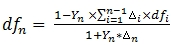The discount factor at time n (dfn) is equal to 1 – Yield at time n (Yn) multiplied by the SUMPRODUCT of all the preceding deltas (?i) and their corresponding discount factors (dfi) divided by 1 + Yield at time n (Yn) multiplied by the delta at time n (?n). If we calculate the time in years (T) for each coupon date as the cumulative time from the spot date to coupon date then the delta for period n is equal Tn – Tn-1.

### Interpolating the par rates

In our #curves table we have swap rates for 1, 2, 3, 4, 5, 7, 10, 15, and 30 years; 9 in total. Those 9 maturities, though, give rise to 60 interest payments each of which requires the calculation of a discount factor which then becomes input (in the numerator of our equation) for the calculation of the next discount factor. In order to make the process work we need to figure out a value for each Yn.
In our example we use the XLeratorDB table-valued function BONDCF to generate all the interest payment dates for the swaps part of the curve then the XLeratorDB aggregate function INTERP to calculate the linearly interpolated Y for each payment date. The XLeratorDB scalar function BUSINESSDATE is used to adjust the payment dates generated by BONDCF to a valid business date in accordance with market convention and the XLeratorDB scalar function YEARFRAC is used to calculate the time in years. The following SQL calculates all the payment dates and the interpolated rates and puts the results in the #wk table which will be used in a subsequent step.
SELECT
N,
date_pmt,
C,
wct.YEARFRAC(LAG(date_pmt,1,@SPOT) OVER (ORDER BY date_pmt),date_pmt,2) as [delta]
INTO
#wk
FROM (
SELECT
k.N,
FROM
(SELECT max(mdate) as maxDate FROM #curves)
CROSS JOIN
#curves c
CROSS APPLY
wct.BONDCF(@SPOT,n.maxDate,0,0,NULL,NULL,2,NULL,NULL,NULL)k
WHERE
c.iType = 'S'
GROUP BY
k.n,date_pmt
)n
WHERE
N.n > 0
The #wk table should contain the following values.
Take note of the fact that the first interest payment date has an interpolated rate of NULL. This is because we are interpolating using the swaps rates and the first swaps interest payment is less than the lower bound of the swaps par rates. In fact, this is exactly the result that we desire as we will calculate the discount factor for the first swaps interest payment from the discount factors in the cash and futures part of the curve. As with the start of the futures strip we use the XLeratorDB aggregate function DFINTERP to get the starting value. However, we are going to restrict the values used in the interpolation to the discount factors in the @cash table that are less than the start of the futures strip and the discount factors in the @futures table that are not interpolated. This becomes the starting value for the swaps calculations.
--Calculate the first coupon date for the swap part of the curve
DECLARE @date_first_coupon as datetime =(SELECT MIN(date_pmt) FROM #wk)

--Calculate the discount factor for the first coupon date
DECLARE @df_first_coupon as float =(
SELECT
wct.DFINTERP(n.mDate,n.df,@date_first_coupon,@SPOT,'df')
FROM (
SELECT mDate, df
FROM @cash
WHERE mdate < @date_start_strip
UNION ALL
SELECT mDate, df
FROM @futures
WHERE iType <> 'I'
)n
)

### The bootstrap calculation

We now have all the information required to calculate the bootstrap values. The bootstrap calculation lends itself very nicely to a Common Table Expression (CTE) except that you cannot use aggregate functions within a CTE and part of the bootstrap calculation requires calculating the sum of the product of the deltas and discount factors for all the previous rows.
One way to overcome this limitation is to build a vector (as a string) of the deltas and discount factors and then use matrix multiplication to do the calculation. If we define D as the vector of deltas and F as the vector of discount factors then the numerator of the bootstrap equation can be express as 1 – Yn * D'F. We can use the XLeratorDB scalar function TCROSSPROD to do the matrix multiplication and add the current delta and the new discount factor to the vectors in the recursive part of the query.
We will create a table variable, @SWAPS, to store the results of the CTE and will join to the #curves table to determine which discount factors are for the maturity dates of the par rates and which are interpolated.
DECLARE @SWAPS as TABLE (
mDate datetime,
iType char(1),
df     float
)

;with mycte as (
SELECT
N
,date_pmt
,@df_first_coupon as df
,CONVERT(VARCHAR(MAX),delta, 128) as deli
,CONVERT(VARCHAR(MAX),@df_first_coupon,128) as dfi
FROM
#wk
WHERE
N = 1
UNION ALL
SELECT
N
,date_pmt
,df
,deli
,dfi + ',' + CONVERT(VARCHAR(MAX),df,128)
FROM (
SELECT
w.N
,w.date_pmt
,@df_spot *(1-w.C*CAST(wct.TCROSSPROD(deli,dfi) as float))/(w.C*w.delta+1)
,m.deli + ',' + CONVERT(VARCHAR(MAX), w.delta, 128)
,m.dfi
FROM
#wk w
INNER JOIN
mycte M
ON
w.n - 1 = m.n
)n(n,date_pmt,df,deli,dfi)
)
INSERT INTO
@SWAPS
SELECT
date_pmt
,CASE
WHEN cRate IS NULL THEN 'I'
ELSE 'S'
END as iType
,df
FROM (
SELECT
date_pmt
,df
,cRate
FROM
mycte
FULL OUTER JOIN
(SELECT * FROM #curves WHERE iType = 'S')n
ON
date_pmt = mDate
)p
The @SWAPS table variable should contain the following values.

### Calculating the continuously compounded zero coupon

In addition to the discount factor, SWAPCURVE calculates the continuously compounded zero rate using this formula: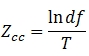### Calculating the zero coupon rate

SWAPCURVE also calculates a zero coupon rate using this formula: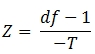### Putting it all together

We can now use the @cash, @futures, and @swaps tables to replicate the output of the SWAPCURVE function. We will select the @cash entries up to the futures start date, the @futures entries up to the swap start date, and the @swaps entries.
SELECT
*
,(df-1)/-T as zero_cpn
,LOG(df)/T as cczero
FROM (
SELECT mDate, df, itype, wct.YEARFRAC(@today,mDate,3) as T
FROM @cash
WHERE mDate < @date_start_strip
UNION ALL
SELECT mDate, df, itype, wct.YEARFRAC(@today,mDate,3)
FROM @futures
WHERE mDate <(SELECT MIN(mdate) FROM #curves WHERE iType = 'S')
UNION ALL
SELECT mDate, df, itype, wct.YEARFRAC(@today,mDate,3)
FROM @SWAPS
)n
ORDER BY 1
This produces the following result.
It was quite straightforward to turn either the continuously compounded zero coupon rate back into a discount factor: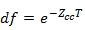or the zero coupon rate back into a discount factor.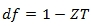Of course, the zero coupon rate produced by SWAPCURVE should not be confused with the spot rate curve.

### Deconstructing the swap curve

While this article is about constructing a yield curve it's actually quite interesting to deconstruct the curve. In other words, given the output of the swap curve, can we figure out what the original inputs were?
To find out how to do that, you'll have to read the second article on this topic which should be out by October 7th.
If you are interested in taking advantage of the powerful tools in the XLeratorDB library of functions, you should download the 15-day trial today. If you are not a SQL Server user you should try out the XLeratorDLL library of functions, which also has a 15-day trial. Download that and see how easy it is to incorporate this functionality into your .NET code.
Got questions? Send us an e-mail at support@westclintech.com or directly to me at cflock@westclintech.com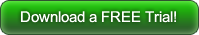Tags:
Categories:
Location: BlogsThe WestClinTech Blog

## Search Blogs

 KeywordsPhrase

## Blog Archives

Archive
 < September 2023 >
SunMonTueWedThuFriSat
272829303112
3456789
10111213141516
17181920212223
24252627282930
1234567
Monthly
Go

### SupportCopyright 2008-2023 Westclintech LLC         Privacy Policy        Terms of Service